View Questions

Exercise 1

Area of the loop, A = 4 cm x 4 cm = 16 x 10- 4 m2
Magnetic field, B = 5 mT = 5 x 10- 3 T
Angle, θ = 00
Magnetic flux through the loop, Φ = BA cos θ = 5 x 10- 3 x 16 x 10- 4 cos 0
= 8 x 10- 6 Wb

Exercise 2

Area of the loop, A = 2 cm2 = 2 x 10- 4 m2
Initial magnetic flux density, B1 = 0.02 Wb/m2
Final magnetic flux density, B2 = 0

The induced emf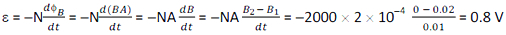Exercise 3

The potential difference between the ends of the axle of a car is given by ε = Bvvl = 3.0 x 10- 5 x 20 x 1.5 = 9.0 x 10- 4 V

Exercise 4

The potential difference between the centre and the end of the blade is given by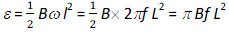Exercise 5

Peak emf is given by
ε0 = NBAω
N = 100, B = 0.10 T, A = 10 cm2 = 10 x 10- 4 m2, ω = 100 rad/s
So, ε0 = NBAω = 100 x 0.10 x 10 x 10- 4 x 100 = 1 V
Peak current through the external resistance = ε0 / (10 + 10) = 1/20 = 0.05 A

Exercise 6

Emf is given by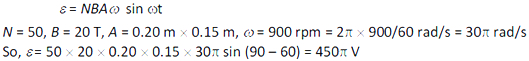Exercise 7

The coefficient of self-induction of a solenoid is given byExercise 8

Emf induced is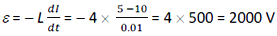Exercise 9

Flux linkages with the winding are
NΦ = LI
So, the self inductance of the winding is
L = NΦ / I
Enegry stored in the winding is given by
U = ½ LI2 = ½ (NΦ / I) I2 = ½ NΦI = ½ NΦV/R = ½ x 1000 x 0.01 x 200/20 = 50 J

Exercise 10

The induced emf in the second coil is given by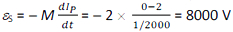Exercise 11

For co-axial solenoids, the mutual inductance is given byThe induced emf in the inner coil is given by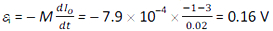Exercise 12

1. Emf produced = Bvl = 0.3 x 0.01 x 0.08 = 2.4 x 10- 4 V
Induced emf will last for the time in which the loop comes out of the field. In this process it will move a
distance equal to the length of shorter side. So, time duration
t = length/speed= 2cm/1cm/s = 2 s

2. Emf produced = Bvl = 0.3 x 0.01 x 0.02 = 0.6 x 10- 4 V
Induced emf will last for the time in which the loop comes out of the field. In this process it will move a
distance equal to the length of longer side. So, time duration
t = length/speed= 8cm/1cm/s = 8 s

Exercise 13

The magnetic flux passing through the loop is given by ΦB = NBA cos ωt = NB πr2 cos ωt

The induced emf,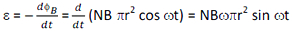Maximum emf is, ε0 = NB ωπr2 = 20 x 3 x 10- 2 x 50 x π (0.08)2 = 0.603 V

Average of emf ε = NB ωπr2 sin ωt over a cycle is zero.

Maximum induced current is,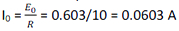Average power is, Pav = ½ ε0I0 = ½ x 0.603 x 0.0603 = 0.018 W

The power dissipated as heat in the coil is supplied by the external mechanism rotating the coil as this mechanism has to do work against the torque produced by induced current which oppose the rotation of the coil.

Exercise 14

Flux linkages with the other coil are
N Φ = MI1
So, change is flux linkages with the other coil are
Δ (NΦ) = MΔ I1 = 1.5 x (20 – 0) = 30 Wb

CBSE Electromagnetic Induction ( With Hint / Solution)
Class XII (By Mr. Ashis Kumar Satapathy)
email - [email protected]